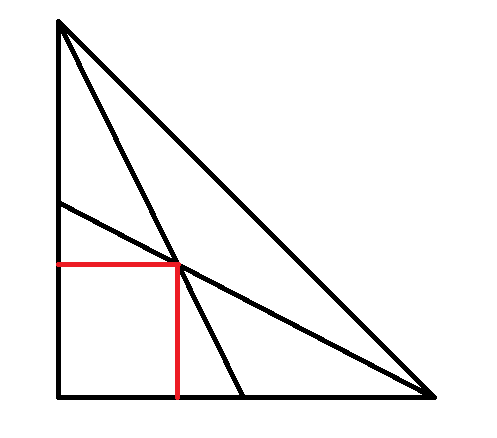# Cut Right Triangle Problem

So, the other day, i was programming something and got a geometry doubt.I apologize for the bad drawing. But anyway, this is a 90-45-45 triangle with legs of 1 unit. It is cut from the 45° point to the exact middle of each of the legs. The red lines are perpendicular to the legs and extends till the meeting point of both the cuts. What is the length of the red lines (only one of them as they form a square) ?

(diagram not to scale)Note by Akshaj Gopalakrishnan
2 years, 4 months ago

This discussion board is a place to discuss our Daily Challenges and the math and science related to those challenges. Explanations are more than just a solution — they should explain the steps and thinking strategies that you used to obtain the solution. Comments should further the discussion of math and science.

When posting on Brilliant:

• Use the emojis to react to an explanation, whether you're congratulating a job well done , or just really confused .
• Ask specific questions about the challenge or the steps in somebody's explanation. Well-posed questions can add a lot to the discussion, but posting "I don't understand!" doesn't help anyone.
• Try to contribute something new to the discussion, whether it is an extension, generalization or other idea related to the challenge.

MarkdownAppears as
*italics* or _italics_ italics
**bold** or __bold__ bold
- bulleted- list
• bulleted
• list
1. numbered2. list
1. numbered
2. list
Note: you must add a full line of space before and after lists for them to show up correctly
paragraph 1paragraph 2

paragraph 1

paragraph 2

[example link](https://brilliant.org)example link
> This is a quote
This is a quote
    # I indented these lines
# 4 spaces, and now they show
# up as a code block.

print "hello world"
# I indented these lines
# 4 spaces, and now they show
# up as a code block.

print "hello world"
MathAppears as
Remember to wrap math in $$ ... $$ or $ ... $ to ensure proper formatting.
2 \times 3 $2 \times 3$
2^{34} $2^{34}$
a_{i-1} $a_{i-1}$
\frac{2}{3} $\frac{2}{3}$
\sqrt{2} $\sqrt{2}$
\sum_{i=1}^3 $\sum_{i=1}^3$
\sin \theta $\sin \theta$
\boxed{123} $\boxed{123}$

Sort by:

I am getting the lenghts to be $\displaystyle \frac{1}{3}$

- 2 years, 4 months ago

Bhai muje apni I'd de sakta hai plzz..

- 1 year, 6 months ago

kyon kya kaam hai?? also, do I know you??

- 1 year, 6 months ago

Na, muje 1 course ke aaghe ke chapter dekhne the or ye free ni hai tab.

- 1 year, 6 months ago

main premium nhi hun, aur main course nhi dekhta…..

- 1 year, 6 months ago

OK thanks BTW :D

- 1 year, 6 months ago

Simply use co-ordinate geometry.....

- 2 years, 4 months ago

Could you please explain that? I am really interested.

- 2 years, 4 months ago

Here is an outline of my method.....

Consider the origin to be the right angled vertex. The remaining two points are located on $\left(1,0\right)\ \&\ \left(0,1\right)$
Hence, the midpoints will be located at $\left(\frac{1}{2},0\right)\ \&\ \left(0,\frac{1}{2}\right)$
Now, simply write the equations of the lines joining the points and you'll arrive at the answer......

- 2 years, 4 months ago

Thanks!

- 2 years, 4 months ago

You are welcome!!

- 2 years, 4 months ago

You can also use similar triangles. Let the length of the red lines be $x$. Consider the right triangle with the vertical red line as it's vertical leg. This right triangle has height $x$ and base length $1 - x$, and is similar to the right triangle with height $1/2$ and base length $1$. By similarity we then have that

$\dfrac{x}{1 - x} = \dfrac{1/2}{1} \Longrightarrow 2x = 1- x \Longrightarrow 3x = 1 \Longrightarrow x = \dfrac{1}{3}$.

- 2 years, 4 months ago